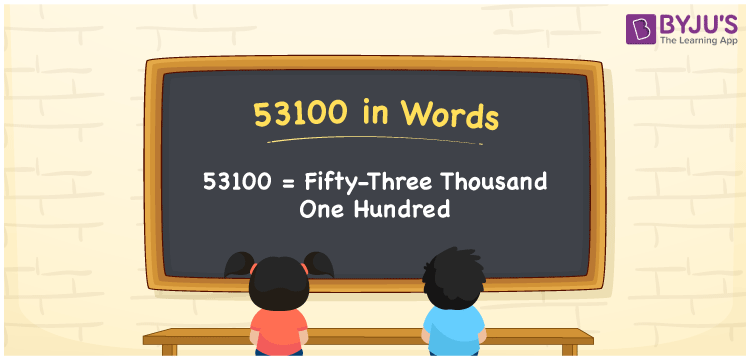# 53100 in Words

53100 in words is expressed as Fifty-Three Thousand One Hundred. For instance, Ajay purchased a two-wheeler electric bike worth Rs. 53100, then you can say, “Ajay purchased a two-wheeler electric bike worth Rupees Fifty-Three Thousand One Hundred”. 53100 is a cardinal number since it denotes an exact quantity. This article explains how to write the number name for 53100 in a precise manner.

 53100 in Words Fifty-Three Thousand One Hundred Fifty-Three Thousand One Hundred in numerical form 53100

## 53100 in English Words

We generally write the numbers in words with the help of the English alphabet. Hence, we can read the number 53100 in words as Fifty-Three Thousand One Hundred.## How to Write 53100 in Words?

53100 is a five-digit number. Hence we need a table of 5 columns. The below table represents the place value chart for the number 53100.

 Ten-Thousands Thousands Hundreds Tens Ones 5 3 1 0 0

Therefore, we can write the expanded form as:

5 x Ten Thousand + 3 x Thousand + 1 x Hundred + 0 x Ten + 0 x One

= 5 x 10000 + 3 x 1000 + 1 x 100 + 0 x 10 + 0 x 1

= 50000 + 3000 + 100 + 0 + 0

= 50000 + 3000 + 100

= 53100

= Fifty-Three Thousand One Hundred

Hence, 53100 in words is written as Fifty-Three Thousand One Hundred

Interesting way of writing 53100 in words

5 = Five

53 = Fifty-Three

531 = Five Hundred and Thirty-One

5310 = Five Thousand Three Hundred Ten

53100 = Fifty-Three Thousand One Hundred

Thus, the word form of the number 53100 is Fifty-Three Thousand One Hundred

53100 is a natural number that is the successor of 53099 and the predecessor of 53101

• 53100 in words – Fifty-Three Thousand One Hundred
• Is 53100 an odd number? – No
• Is 53100 an even number? – Yes
• Is 53100 a perfect square number? – No
• Is 53100 a perfect cube number? – No
• Is 53100 a prime number? – No
• Is 53100 a composite number? – Yes

## Frequently Asked Questions on 53100 in Words

Q1

### Write 53100 in words.

53100 in words is Fifty-Three Thousand One Hundred.
Q2

### Simplify 50000 + 3100, and express in words.

Simplifying 50000 + 3100, we get 53100. Therefore, the number 53100 in words is Fifty-Three Thousand One Hundred.
Q3

### Is 53100 a composite number?

Yes, the number 53100 is a composite number.